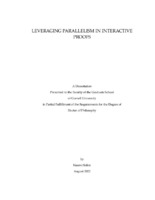## Leveraging Parallelism in Interactive Proofs##### Abstract
Interactive proof systems enable one party (the prover) to convince another (the verifier) of the validity of some computational statement, and ensure that the verifier will be convinced if and only if the statement is true. Since their introduction, interactive proofs have become one of the most fundamental concepts in theoretical computer science, and have led to breakthrough results across complexity theory and modern cryptography. In recent years, there has been immense interest in succinct proof systems, which enable the verifier to certify computations much faster than computing them. However, the efficiency of these proof systems, specifically the time required to generate a proof, remains a major bottleneck between theory and practice. In this thesis, we approach this challenge through the lens of parallelism. We first consider whether the aforementioned bottleneck can be overcome by leveraging access to parallel processors.In existing proof systems, the time to generate a proof scales at least polylogarithmically with the complexity of the statement being proven–that is, proving correctness of a T-time computation requires time T * polylog(T). In this thesis, we introduce a new paradigm for proof systems in which the prover uses only polylog(T) processors to both compute and prove a T-time computation in time T + polylog(T)–that is, near-optimal parallel time allowing only a small additive overhead. We call such a system a SPARK, or a SPARG when proving only deterministic computations, and show the following: - Constant-round SPARKs for NP from collision-resistant hash functions. - Non-interactive SPARKs for NP from collision-resistant hash functions and succinct non-interactive arguments of knowledge. - Non-interactive SPARGs for P from LWE. Finally, we focus on proof systems for a specific task–that of efficiently verifying that a "long" computation was done correctly. This is captured by the notion of a Verifiable Delay Function (VDF). We obtain the first construction of a VDF in the plain model that relies only on the minimal assumption of a sequential function, along with standard assumptions, by showing that SPARGs for P imply VDFs. In the latter part of this thesis, we introduce a new type of VDF that is more amenable to distributed settings, called a Continuous VDF, and give a construction in the random oracle model based on the repeated squaring assumption. As an additional contribution, we show that the hardness of computing Nash equilibria can be based on the existence of Continuous VDFs.
368 pages
2022-08
##### Keywords
interactive proof; succinct argument; verifiable delay function
Pass, Rafael N.
##### Committee Member
Shi, Runting
Kleinberg, Robert David
Computer Science
##### Degree Name
Ph. D., Computer Science
##### Degree Level
Doctor of Philosophy